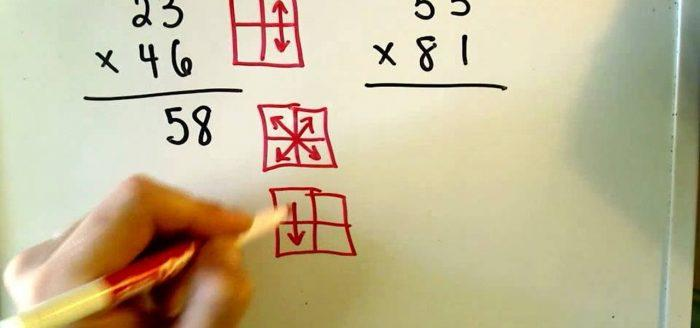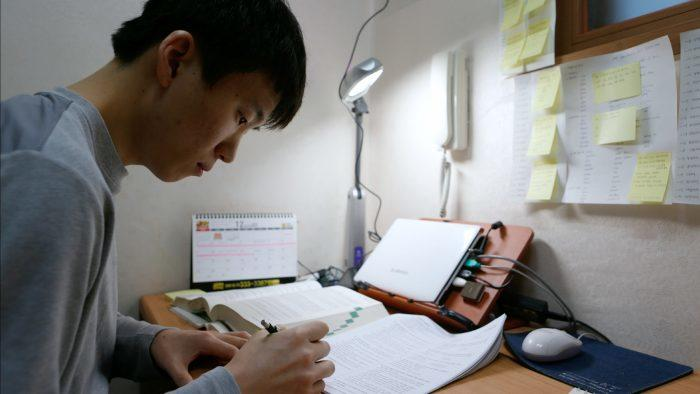Do you find algebra annoying? Probability pedestrian? Trigonometry tedious? Did you hate homework at school? It sometimes seems it's easier to hate maths than to love it. But never, fear, there are many tricks to improving your maths skills.

With things like theories, algebraic formulae, geometry, solving equations with multiple variables, maths sometimes can be a bit of a minefield and lots of student's try hard to improve their mental maths skills.

However, a lot of people develop a dislike for maths solely because they struggle with mental arithmetic.

Young learners tend to hate it! So much so that they can barely bring themselves to open their textbook. They struggle with addition and subtraction and before they've even fully grasped it, they're having to take exams where they're tested with activities on the multiplication and division of whole numbers.

Stay strong, you needn't give up! If you go back to learning the basics of addition, multiplication, division and square roots, it'll really help you out in your daily life.

Maths is built on rational thinking. You're probably better than it than you think. If you've ever redecorated a room, you'll have used a bit of algebra and geometry without even knowing!

Don’t you find it weird that you use the calculator on your phone to do something as simple as working out your bill at a restaurant or for subtracting the discount during the sales?

It may seem that technology has taken away our ability to work things out in our head. However, that’s just a myth! It's just a tool to help us, not hinder us.

Anyone can learn to count quickly.

Here is what you should know about math tutoring Toronto.You can learn to do sums really quickly, too! (Source: WonderHowTo)

How do you get better at maths? You don't need to enrol in a four-year course. In fact, it'll only take a fraction of your time.

In this article, we've got a few tips and tricks that will help you count more quickly. Don't worry, we're not asking you to do complex calculus, word problems, or quadratic and linear equations, just showing you how to solve problems in your head! Step 1...

Discover a mental math class here.Mansur
Mathematics Teacher4.86 (7) \$30/h
First lesson is free!Abraham
Mathematics Teacher5.00 (4) \$30/h
First lesson is free!Zach
Mathematics Teacher5.00 (9) \$18/h
First lesson is free!Mahshid
Mathematics Teacher5.00 (6) \$30/h
First lesson is free!Safa ur
Mathematics Teacher
\$16/h
First lesson is free!Huakai
Mathematics Teacher
\$15/h
First lesson is free!Abhilash
Mathematics Teacher
\$14/h
First lesson is free!Tai
Mathematics Teacher5.00 (7) \$30/h
First lesson is free!

## Recap the Basics of Mental Arithmetic

Go back to addition, multiplication, and division tables, and learn the square roots (up to 12 or 15).

There are also techniques to easily do sums and times tables with the digits from 1 to 9. All you have to do is a bit of rounding and then you'll be adding and subtracting like nobody's business.

### Here are a few maths tricks for fast calculation:

• To add numbers ending in 9, add the next number then subtract 1.
• For example, to work out 525 + 29, do 525 + 30 - 1 = 555 - 1 = 554.
• To add 11, it’s the same process. Just add the tens, then add 1. For example, 428 + 11 = 428 + 10 + 1 = 438 + 1 = 439.
• Do the same thing for numbers that end in 1. For example, 668 + 31 = 668 + 30 + 1 = 698 + 1 = 699.
• To multiply by 4, you just have to double the number, then double it again. For example, 36 x 4 = 36 x 2 x 2 = 72 x 2 = 144.
• Multiplying by 10 is really easy, especially when talking about integers. All you do is add a 0 to the end of the number. For example, 128 x 10 = 1280.
• When there’s a decimal point, you just have to move the decimal one digit to the right. For example, 68.5 x 10 = 685.
• To multiply by 100, you just add 2 zeros to the end of the number or move the decimal place 2 digits to the right, etc.
• To multiply by 5, you can multiply by 10 then divide by 2. For example, 224 x 5 = 224 x 10 / 2 = 2240 / 2 = 1120.

## Mental Maths Practice Makes Perfect

These useful tips in maths are great since they exploit how our brain works. When used in the right way, your brain is capable of incredible complex reasoning.

However, our brain is also kind of lazy. It's constantly looking to do the most work with the least amount of effort.

We live in a world that’s constantly going faster and faster. Everything in our daily lives is instant. We want the dishes to be done before we start the machine. Our clothes to be washed and ironed for us and the dinner to be made before we get home. Our brain wants the same when it comes to mental arithmetic!

By mastering arithmetic, you're simplifying your life, improving your reactions, and gaining mathematical reflexes that a ninja would be jealous of.

Find online math courses here.With a good tutor you'll quickly learn to do maths without even thinking! (Source: IDN Times)

Look for good maths tutors if you want maths help to learn these reflexes! A private tutor might be just what the doctor ordered.

Depending on your level and skill, as well as your weaknesses and your expectations, you’ll probably need tailored tutoring programmes if you're looking to surpass your classmates.

A tutor can show you how to use the aforementioned tips and tricks and help you develop other skills to improve your mental arithmetic.Mansur
Mathematics Teacher4.86 (7) \$30/h
First lesson is free!Abraham
Mathematics Teacher5.00 (4) \$30/h
First lesson is free!Zach
Mathematics Teacher5.00 (9) \$18/h
First lesson is free!Mahshid
Mathematics Teacher5.00 (6) \$30/h
First lesson is free!Safa ur
Mathematics Teacher
\$16/h
First lesson is free!Huakai
Mathematics Teacher
\$15/h
First lesson is free!Abhilash
Mathematics Teacher
\$14/h
First lesson is free!Tai
Mathematics Teacher5.00 (7) \$30/h
First lesson is free!

## Update Your Brain to Improve Mental Maths

Even though the computing comparison may seem trivial, your brain does work like a computer.

According to the Salk Institute in La Jolla, California, our brain has a minimum capacity of around 1 petabyte (1,000GB). This is around the same capacity as everything on the Internet!

The researchers at this American institute discovered that our hippocampus (the gland at the base of our brain) and its neurons function at 100% capacity by using very little energy.

If our brain is the core unit with a hard drive, our skills and knowledge is the operating system. You should keep them up to date, right?

Our brain needs change to learn and activate its various faculties. You should make it use plenty of neurons so that information moves around more quickly. This is how you learn to calculate more quickly both in your private maths tutorials and in your daily life.

Use it to get better at maths, "install" new programmes, learn new methods to improve, and do past papers, worksheets, and even geometric puzzles.

Maths tutorials are perfect for this. Whether online, at university, college, secondary school, or even private maths tutorials, you’ll always find new ways to do mental arithmetic.

## Don’t Rush Maths

Don’t run before you can walk. The same can be said for maths. You can learn maths in many different ways so don’t waste your time with methods that don’t work. Stay calm.

Whether you want to improve your mental arithmetic in private maths tutorials with a qualified mathematician or solve maths problems quickly, start slowly and take your time. Consolidate your knowledge with regular maths exercises and keep practicing.

Don’t rush! This usually leads to mistakes and accidentally skipping essential steps, and, inevitably, bad marks.It's definitely more haste, less speed when it comes to maths. (Source: CreativINDIE)

Start slowly, deconstruct the problem bit by bit, see what you need to do, and how you can do it.

Call upon your knowledge to see if you can find a solution.

It's better to find an answer quickly. It’s important to know the route you need to take to find the answer. A teacher will know how to identify the problem and the approach you’ll need to take.

Take your time learning the basics of arithmetic, the mathematical theories, and apply them in easy exercises.

Gain confidence in the beginning and then increase the difficulty of each exercise.

This is how you advance and get better at maths.

## Have Fun With Maths Lessons

Even if a teacher is the best person to identify a student’s difficulties and rectify them, you should know that you can (with the help of your parents and friends) get better at maths.

Daily life is full of situations where you can make progress. Make sure to use them in a fun way and enjoy them.

The best way to progress is to enjoy what you’re doing.

Try adding up the cost of your shopping at the supermarket or work on some math puzzles or games.

This doesn’t mean counting everything to the penny. You can quickly work it out using some quick mental arithmetic.

Another way to improve your speed in maths classes is cooking. To make a cake you develop your mental arithmetic by working with large numbers and converting the units of measurement (grams, kilos, litres, etc.).How do you improve the best recipe? Add a sprinkle of maths, of course! (Source: The Imagination Tree)

This is your chance to work with proportionality and fractions by working out how to cut a cake for 4 people into 6 equal parts, for example.

Maths surrounds us constantly: whether it’s working out how long a car journey will be, the number of kilometres, doing some DIY, or sewing, you’ll always find a fun way to practise it.

There aren’t 100 ways to get better at maths: just training, repetition, and activities to practice getting quicker and quicker in your calculations.

Whether you’re working with a tutor or not, you need to train your brain and work on your mathematical reflexes.

The brain is a muscle, it needs to be trained and fed in order to get the most out of it. After time you’ll stop noticing that your calculations are getting faster and faster. The student becomes the teacher!

Need a teacher Mathematics ?5.00/5, 1 votesLoading...